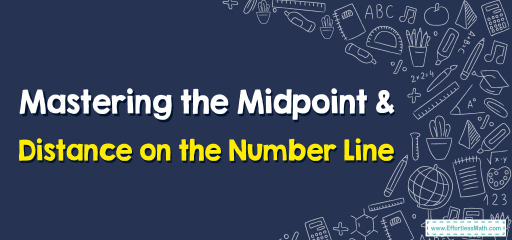# Mastering the Midpoint & Distance on the Number Line

In geometry, lines have starting and ending points. Between these points, we can find the exact middle, called the midpoint, and measure how long the line is, known as the distance. This guide will explain these two concepts in simple terms. So, if you're looking to understand how to find the middle of a line or how long it is, you're in the right place!## Step-by-step Guide: Distance and Midpoint on the Number Line

Understanding the Midpoint:
The midpoint between two numbers (or points) on a number line is the number that lies exactly halfway between them. The formula to find the midpoint $$M$$ between two points $$A(x_1)$$ and $$B(x_2)$$ is:
$$M = \frac{x_1 + x_2}{2}$$

the Concept of Distance:
The distance between two numbers (or points) on a number line is the absolute difference between them. The formula to find the distance $$D$$ between two points $$A(x_1)$$ and $$B(x_2)$$ is:
$$D = |x_2 – x_1|$$

### Examples

Example 1: Midpoint
Find the midpoint between the numbers $$4$$ and $$10$$ on the number line.

Solution:
Using the midpoint formula:
$$M = \frac{4 + 10}{2}$$
$$M = \frac{14}{2}$$
$$M = 7$$
So, the midpoint is $$7$$.

Example 2: Distance
Find the distance between the numbers $$-3$$ and $$5$$ on the number line.

Solution:
Using the distance formula:
$$D = |5 – (-3)|$$
$$D = |5 + 3|$$
$$D = 8$$
So, the distance is $$8$$ units.

### Practice Questions:

1. Find the midpoint between the numbers $$2$$ and $$8$$ on the number line.
2. Calculate the distance between the numbers $$-7$$ and $$3$$ on the number line.
3. If the midpoint between two numbers $$a$$ and $$b$$ is $$5$$, what is the value of $$b$$ given $$a = 3$$?

1. The midpoint is $$5$$.
2. The distance is $$10$$ units.
3. The value of $$b$$ is $$7$$.

### What people say about "Mastering the Midpoint & Distance on the Number Line - Effortless Math: We Help Students Learn to LOVE Mathematics"?

No one replied yet.

X
30% OFF

Limited time only!

Save Over 30%

SAVE $5 It was$16.99 now it is \$11.99